# Motion and Time NCERT Solutions Class 7

In this page we have NCERT Solutions for Motion and Time Class 7 science CBSE . Hope you like them and do not forget to like , social share and comment at the end of the page.

Question 1

Classify the following as motion along a straight line, circular or oscillatory motion:
(i) Motion of your hands while running.
(ii) Motion of a horse pulling a cart on a straight road.
(iii) Motion of a child in a merry-go-round.
(iv) Motion of a child on a see-saw.
(v) Motion of the hammer of an electric bell.
(vi) Motion of a train on a straight bridge.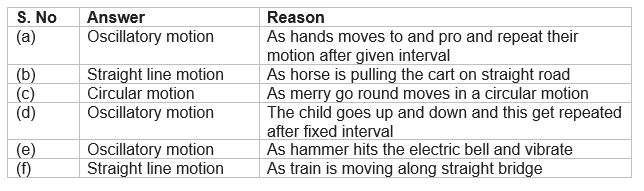Question 2
Which of the following are not correct?
(i) The basic unit of time is second.
(ii) Every object moves with a constant speed.
(iii) Distances between two cities are measured in kilometers.
(iv) The time period of a given pendulum is not constant.
(v) The speed of a train is expressed in m/h.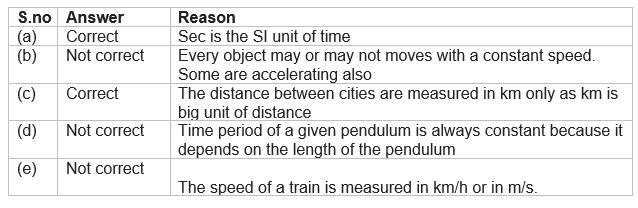Question 3
A simple pendulum takes 32 s to complete 20 oscillations. What is the time period of the pendulum?
Numbers of oscillations = 20
Time taken = 32 sec
Time period of the pendulum =Time taken/Numbers of oscillations = 32/20 = 1.6 s.

Question 4
The distance between two stations is 240 km. A train takes 4 hours to cover this distance. Calculate the speed of the train.
Distance between two stations = 240 km
Time taken = 4 hrs.
Speed of train is given as
= Distance/Time taken = 240/4 = 60 km/hr.

Question 5.
The odometer of a car reads 57321.0 km when the clock shows the time 08:30 AM. What is the distance moved by the car, if at 08:50 AM, the odometer reading has changed to 57336.0 km? Calculate the speed of the car in km/min during this time. Express the speed in km/h also.
Given
Initial reading of odometer = 57321.0 km
Final reading of odometer = 57336.0 km
Initial Time = 8:30 AM
Final Time = 8:50 AM
We know that Odometer measure the distance travelled by the car
So Total distance covered by car= Final reading of odometer - Initial reading of odometer
=57336.0 - 57321.0 = 15.0 km
Total time taken by Car = Final Time - Initial Time =8:50 AM - 8:30 AM = 20 minutes= 20/60 hrs. = 1/3 hrs.
Now Speed of the car is defined as
= Total distance covered/Total time taken = 15.0/20 = 0.75 km/min
Also
Speed of the car in km/hr. =15/ (1/3) = 45 km/hr.

Question 6
Salma takes 15 minutes from her house to reach her school on a bicycle. If the bicycle has a speed of 2 m/s, calculate the distance between her house and the school.
Given
Speed of bicycle = 2 m/s
Time taken = 15 mins = 15 × 60 secs = 900 secs
Now we know that
Speed = Distance/Time
Therefore
Distance = Speed × Time = 2 × 900 meters = 1800 meters = 1.8 km

Question 7.
Show the shape of the distance-time graph for the motion in the following cases:
(i) A car moving with a constant speed.
(ii) A car parked on a side road.
 A car moving with a constant speed covers equal distance in equal line. So it is represented by the give straight line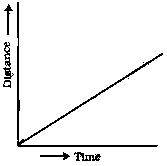A car parked on a side road So it is not moving and no distance is being covered by it. So distance remains constant with time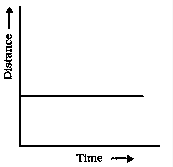Question 8
Which of the following relations is correct?
(i) Speed = Distance × Time
(ii) Speed = Distance/Time
(iii) Speed = Time/Distance (iv) Speed = 1/Distance × Time
(ii) Speed = Distance/Time

Question 9
The basic unit of speed is:
(i) km/min
(ii) m/min
(iii) km/h
(iv) m/s
(iv) m/s

Question 10.
A car moves with a speed of 40 km/h for 15 minutes and then with a speed of 60 km/h for the next 15 minutes. The total distance covered by the car is:
(i) 100 km
(ii) 25 km
(iii) 15 km
(iv) 10 km
(ii) 25 km
Firstly, the car is moving with 40km/h for 15 minutes.
15 minutes = 15/60 hrs. = 1/4 hrs.
Distance covered = 40 × 1/4 = 10 km
Secondly, the car is moving with 60km/h for 15 minutes. Distance covered = 60 × 1/4 = 15 km Therefore
Total distance covered = 10 km + 15 km = 25 km

Question 11
Suppose the two photographs, shown in Fig. 13.1 and Fig. 13.2, had been taken at an interval of 10 seconds. If a distance of 100 meters is shown by 1 cm in these photographs, calculate the speed of the blue car.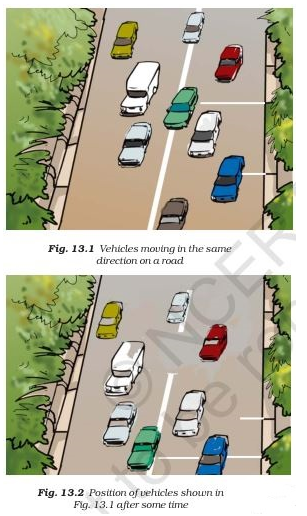First, measure the distance with the help of scale and then proceed as given below.
Suppose the distance measured by scale is 2cm.
Multiply 2 with 100 to get the actual distance = 2 × 100 = 200 m. (1cm = 100m)
Interval time between the photos taken = 10 s.
Speed of the blue car = 200/10 m/s = 20 m/s

Question 12
Below shows the distance-time graph for the motion of two vehicles A and B. Which one of them is moving faster?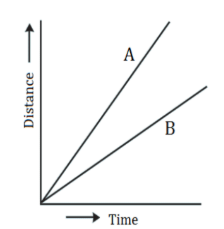Vehicle A is moving faster because it has more slope than vehicle B. In distance-time graph, speed is measured by its slope.

Question 13
Which of the following distance-time graphs shows a truck moving with speed which is not constant?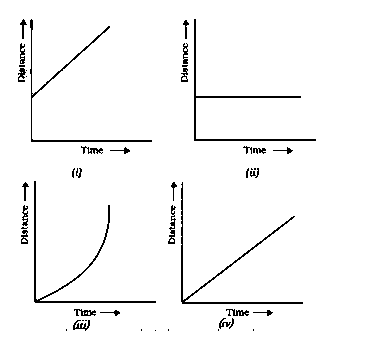Option (iii) because the slope of the graph is not a straight line and hence it does not show a uniform motion.

li>Notes
• NCERT Solutions
• Assignments